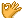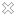•闪存
•博客
• 发言小组
• 投递新闻
• 提问博问
• 添加收藏
•文库

3

1

##10python-flask 框架中的url_for

comments = [] @app.route("/", methods=["GET", "POST"]) def index(): if request.method == "GET": retu
3

1

2

##Python 在sleep期间怎么做其他事？

def times(h=0, h1=0): '''h表示设定的小时，m为设定的分钟''' while True: # 判断是否达到设定时间，例如0:00 while True: now = datet
4

3

##10求助，python中文乱码

import pandas as pd import numpy as np from pandas import DataFrame,Series import os import datetime
3